Home MATHEMATICS TOPIC 4: LOGARITHMS ~ MATHEMATICS FORM 2

# TOPIC 4: LOGARITHMS ~ MATHEMATICS FORM 2

139
0We always ask ourselves, how many of one number do we multiply to get another number? For example; how many 3s do we multiply to get 81? All these kind of questions will be answered in this unit. Make sure you understand. Start reading now……!

Standard Form is also called Scientific Notation. It is a way of writing a number into two parts. For example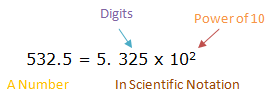• Thedigits(with the decimal point placed after the first digit) followed by
• X 10 to a powerthat puts a decimal point where it should be (i.e. it shows how many places to move the decimal point).

Numbers in Standard Form

Write numbers in standard form
How to write a number in standard form?
To figure out the power of 10, think of how many decimal places to move:
• When the number is 10 or greater, the decimal place has to move to the left and the power of 10 will be positive. For example; 47 055 = 4. 7055 x 104
• When the number is smaller than 1, the decimal point has to move to the right and the power of 10 will be negative. For example;
0.00025 will be written as 2.5 x 10-4
For example; 4.5 would be written as 4.5 x100 we didn’t have to move the decimal point, so the power is 100. But now it is in standard form.
Note that: After putting the number in scientific notation make sure that the digits part is between 1 and 10 (it can be 1 but never 10). And the power part shows exactly how many places to move the decimal point.
Computations which Involved Multiplication and Division of Numbers Expressed in Standard Form
Perform computations which involved multiplication and division of numbers expressed in standard form
Definition of a logarithm
A logarithm answers the question: How many of one number do we multiply to get another number. For example; how many of 2s do we multiply to get 16? Answer: 2 x 2 x 2 x 2= 16 so we needed to multiply 4 of the 2s to get 16. So the logarithm is 4.
How to write it?
We would write the number of 2s we need to multiply to get 16 is 4 as:
The two things are the same: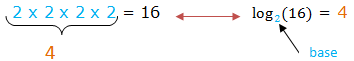The number we are multiplying is called the base. So we can say ‘the logarithm of 16 with base 2 is 4’ or ‘log base 2 of 16 is 4’ or ‘the base-2 log of 16 is 4’.
Not that we are dealing with 3 numbers:
• The base (the number we are multiplying in our example it is 2)
• How many times to use it in multiplication (in our example it is 4 times, which is the logarithm)
• The number we want to get (in our example it is 16)
There is a relationship between the exponents and logarithms. The exponent says how many times to use the number in a multiplication and logarithm tells you what the exponent is. See the illustration below: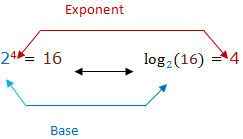Generally: ax = y in logarithmic form is: LogaY = X
Example 1
write the following statements in logarithmic form: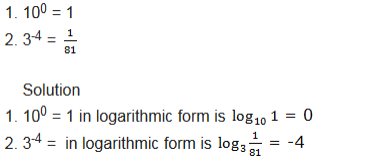The Laws of Logarithms
State the laws of logarithms
There are several laws of logarithms which help in evaluating them. These laws are valid for only positive real numbers. The laws are as follows: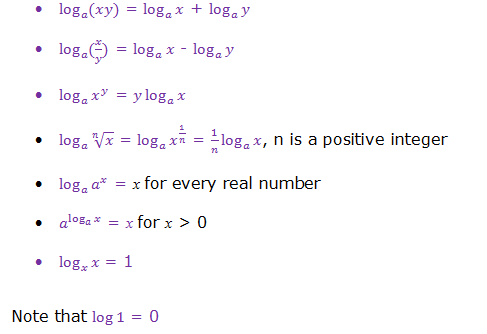Verification of the Laws of Logarithms Using the Knowledge of Exponents
Verify the laws of logarithms using the knowledge of exponents
Activity 1
Verify the laws of logarithms using the knowledge of exponents
The Laws of Logarithms to Simplify Logarithmic Expressions
Use the laws of Logarithms to simplify logarithmic expressions
Example 2
Use the laws of logarithms to evaluate the following: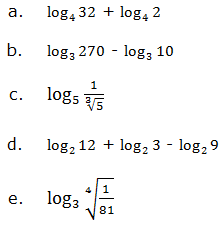Solution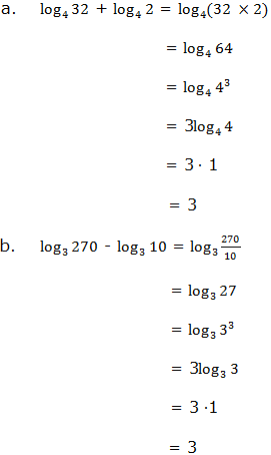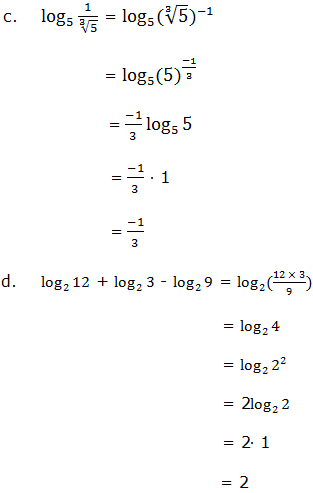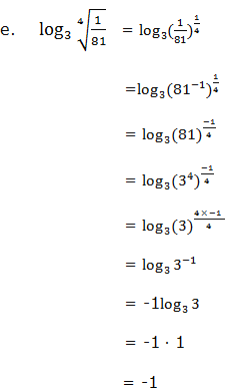Change of base
This is a formula for change of base. For any positive ab (ab ≠0) we have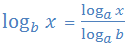Given,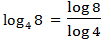then find a number which is a common base to both 8 and 4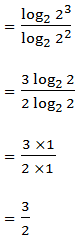Example 3
Solve.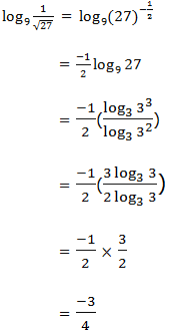Logarithms to Base 10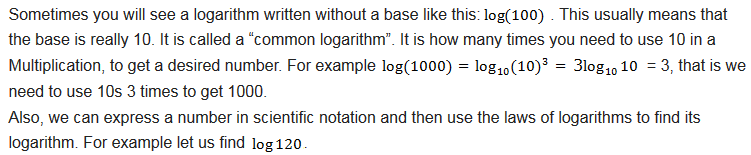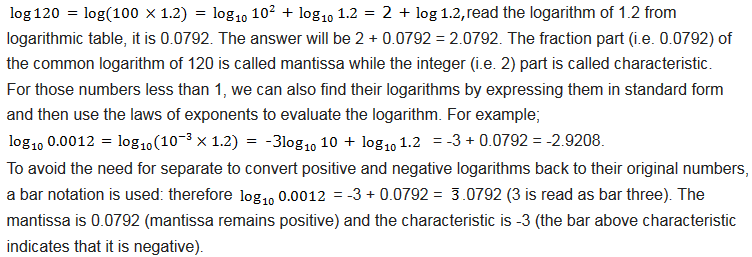Logarithmic Equation
Solve logarithmic equation
Use the laws of logarithms to evaluate the following:SolutionLaws of Logarithms to Find Products, Quotients, Roots and Powers of Numbers
Apply laws of logarithms to find products, quotients, roots and powers of numbers
Here we deal with all 4 operations which are addition, subtraction, multiplication and division. All operations are just as usual operations except division when we are given a negative characteristic. For example;Example 4
evaluate the following:Logarithmic Tables to Find Products and Quotients of Numbers Computation
Apply logarithmic tables to find products and quotients of numbers computation
Most of the logarithmic tables are of base 10 (common logarithms). When we want to read a logarithm of a number from logarithmic table, we first check if the number is between 0 and 10 (but not 0 or 10) because the table consists only of logarithms of numbers between 0 and 1.
For example; what is the logarithm of 5.25 from the table. Our number is between 0 and 1. We look at the most left column and find where 52 is (we ignore the decimal point). Then slide your finger along this row to the right to find column of the next digit in our example is 5. Read the number where the row of 52 meets the column of 5. The logarithm of 5.25 is 0.7202.
If the number has 4 digits like 15.27, we do the following. First of all, checking our number we see that it is greater than 10. The number is between 10 and 100. And we know that the logarithm of 10 is 1 and logarithm of 100 is 2. So logarithm of 15.27 is between 1 and 2, normally less than 2 but greater than 1, hence 1.something. That something we need to find it in a logarithm table. Look at the most left column the row labeled 15, then, slide your finger to the right to find the column labeled 2. Read the number where the row of 15 meets the column of 2, the number is 0.1818. We are remaining with one digit which is 7. If your log table has a part with mean difference table, slide your finger over to the column in that table marked with the next digit of the number you are looking up, in our example it is 7. Slide over to row 15 and mean difference 7. The row of 15 meets mean difference column 7 at number 20. Add the two numbers obtained (the mean difference number is added to the last digits of our first number we obtained) i.e. 0.1818 + 20 = 1838. Now add characteristic which is 1 since 15.27 is between 10 and 100. We get 1 +0.1838 = 1.1838. Therefore Log 15.27= 1.1838.
Note that if you are given a number with more than 4 digits, first round off the number to 4 digits and then go on with similar procedures as explained in examples above.
To find the number whose logarithm is known, we can call it ant-logarithm the same logarithmic table can be used. For example to find the number whose logarithm is 0.7597, look at the central part of the log table find the number (mantissa) 7597. This is in the intersection of the row labeled 57 and column 5. So the number is 575. But in order to get correct answer we have to consider characteristic of our logarithm which is 0. This means our number is between 0 and 10 because the numbers whose logarithms are 0.something are between 0 and 10. Hence, we need to place one decimal point from left to our number to make it be between 0 and 10. Therefore the number will be 5.75 i.e. log 5.75 = 0.7597, thus antilog 0.7597= 5.75.
How to find the ant-log
Step1: Understand the ant-log table. Use it when you have log of a number but not the number itself. the ant-log is also known as the inverse log.
Step 2: Write down the characteristic. This is the number before decimal point. If you are looking up the ant-log of 2.8699, the characteristic is 2. Remove it from the number you are looking up. But never forget it because it will be used later. So it is better if you write it somewhere.
Step 3: Find the row in the most left column that matches the first two numbers of the mantissa. Our mantissa is 8699. So run your finger down that column until you find .86.
Step 4: Slide your finger over to the column marked with the next digit of the mantissa. For 2.8699, slide your finger along the row marked .86 to find the intersection with column 9. This reads 7396. Write this down.
Step 5: If your ant-log table has a table of mean difference, slide your finger over to the column in that table marked with the next digit of the mantissa. Make sure to keep your finger in the same row. Considering our example, slide your finger over the to the last column in the table, column 9. The intersection of row .86 and mean difference column 9 is 15. Write it down.
Step 6: Add the two numbers obtained from the two previous steps. In our example, these are 7396 and 15. Adding them i.e. 7396 + 15 = 7411.
Step 7: Use characteristic to place decimal point. Our characteristic is 2, which means our answer is between 100 and 1000 because log 100 = 2 and log 1000 = 3. For the number 7411 to fall between 100 and 1000, the decimal point should be placed after 3 digits. So, the final answer is 741.1 therefore the ant-log of 2.8699 is 741.1.
Example 5
Find the product of 25.75 ×450.
Solution;
From Logarithmic laws we saw that multiplication of two numbers is the same as addition of two the same two numbers. How to do it?
Let x = 25.75 ×450
log x = log (25.75 ×450)
which is the same as
now, you can read log of your numbers from the logarithmic table as we learnt in the previous lesson, you will find:
log 25.75 = 1.4108 and log 450 = 2.6532
thus, log x = 1.4108 + 2.6532
log x = 4.0640
in order to obtain the value of x we have to find the inverse log of 4.0640 or ant-log of 4.0640
so, x = ant-log 4.0640
x = 11590
therefore, 25.75 ×450 = 11590
always the logarithmic calculations are set out in tabular form to make the solution not too long as above.
If we set our example in tabular form it will look like this: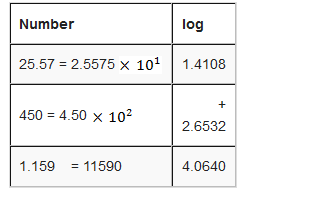Logarithmic Tables to Find Roots and Power of Numbers
Apply logarithmic tables to find roots and power of numbers
Example 6
Calculate using logarithms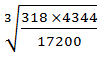Solution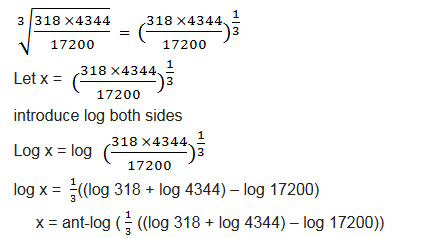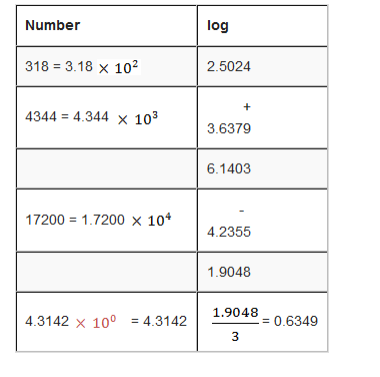Exercise 1
1. Write each of the following in standard form:
1. 167200
2. 0.00235
3. 245.750
4. 45075
2. Write each of the following in decimal numerals: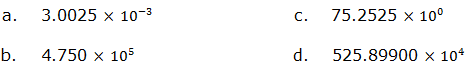3. Calculate value of x for each of the following: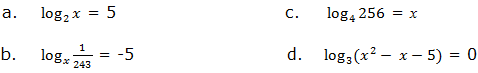4. Simplify each of the following without using logarithmic table:5. Given that log 2 = 0.3010, log 3 = 0.4771 and log 7 = 0.8451, find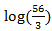6. Find log of each of the following numbers using logarithmic table:
1. 500
2. 0.00135
3. 52. 25
4. 895000
7. Find the value of x for each of the following numbers:
1. Log x = 2.8751
2. Log x = 1.8186
8. Find the value of each of the following expressions: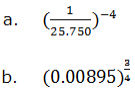10. Determine the number whose
1. Logarithm in base 10 is -3
2. Logarithm in base 5 is 3